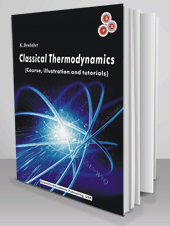﻿ Scientific & Academic Publishing: Books## Classical Thermodynamics (Course an tutorials)

• K. Boubaker is an Associate Professor from University of Tunis. His domains of interest: Applied Physics, Heat Transfer, Biophysics, Modelling, Semiconductors, Renewable Energies and Numerical Analysis.
• ISBN: 978-0-9838996-0-0

Description

In 1730, Daniel Bernoulli described a "random motion" of molecules of a gas with chocks between them and with the container walls. Two centuries later this “thermal agitation motion” became a fundament of classical thermodynamics.

This book, ‘Classical Thermodynamics’, tries to present the whole set of fundaments, involving Gas Kinetic Theory, thermodynamic laws and relevant notions like Temperature, Entropy and Free Enthalpy.

A complete tutorial is presented along with tangible, interesting and useful application. Original and rich illustrations are also provided for both beginners and experts.

CHAPTER 1.

GAS KINETIC THEORY

1. Preliminary

1.1. Historic Preview

1.2. Fundamental Hypotheses

2. Principles

2.1. Boltzmann Statistic Mechanics

2.1.1. Definitions, Phase Space and Domains

2.1.2. Boltzmann Fundamental Hypotheses, State Probability

2.2. Monatomic Ideal Gases Case Features

3. Applications of the Kinetic Theory of Gases

3.1. Ideal Gas Pressure

3.2. Number of Hitting Molecules Per Unit Time

CHAPTER 2.

INTRODUCTION TO THERMODYNAMICS HISTORIC PREVIEW

1. Definitions

1.1. A Thermodynamic System

1.2. States, Equations of State, Representations

1.2.1. Thermodynamics Variables (Variables of State)

1.2.2. Equilibrium States

1.2.3. Equation of State and Function of State

1.2.3.1. Equation of State

1.2.3.2. Function of State

1.2.4. Representations

1.2.4.1. Clapeyron Representation: (P, V) Diagram

1.2.4.2. Amagat Representation : (PV, P) Diagram

2. System Transformations

2.1. Quasi-Static Transformations

2.2. Reversible Transformations

2.3. Irreversible Transformations

2.3.1. Gas Relaxation

2.3.2. Spontaneous Heat Transfer

3. Some Particular Transformations

4. The Notion of Temperature

4.1. Zeroth Law of Thermodynamics

4.2. Thermometry and Thermometric Standards

4.2.1. Experimental Facts, Thermometry Fixed Points

4.2.2. Legal Temperature Scale

4.2.3. Other Temperature Scales

CHAPTER 3.

THE FIRST LAW OF THERMODYNAMICS

1. Work

1.1. Mechanical Work

1.1.1. General Expression

1.1.2. Case of a Quasi-Static or Reversible Transformation of a Fluid

1.2. Mechanical Work Graphic Representation

1.3. Some Particular Transformations

1.4. Work Measurement Unity

2. Heat (Calorific Energy)

2.1. Sensible Heat

2.2. Phase Change Latent Heat

2.3. Mixture Law

2.4. Heat Transfers Study

2.4.1. Conduction

2.4.2. Convection

2.4.2.1. The Natural Convection

2.4.2.2. Forced Convection

2.5. Units

CHAPTER 4.

WORK AND ENERGY

3. Principle of Heat-Work Equivalence

4. Consequence of the Principle of Equivalence: The First Principle

4.1. Internal Energy

4.1.1. Microscopic Aspect

4.1.2. Macroscopic Aspect

4.2. First Principle Differential Expression

5. Enthalpy Function H

5.1. Differential Expression of the Enthalpy

5.2. Homogeneous Fluid Case

6. Application of the First Principle in Homogeneous Fluids: Calorimetric Coefficients

6.1. Calorimetric Coefficients

6.1.1. Coefficients CV, CnV, CmV and l

6.1.2. Coefficients CP, CnP, CmP and h

6.1.3. Coefficients λ and µ

7. Calorimetric Coefficients Relation

7.1. Isochoric Transformation

7.2. Isobar Transformation

CHAPTER 5.

IDEAL GASES-REAL GASES A MICROSCOPIC APPROACH

1. Ideal Gases

1.1. Ideal Gases Equation of State

1.1.1. Boyle-Mariotte Law

1.1.2. Charles and Gay-Lussac First Law

1.1.3. Charles and Gay-Lussac Second

1.1.4. Equation of State

1.2. Ideal Gases Diagrams

1.2.1. Clapeyron Diagram

1.2.2. Amagat Diagram

1.3. Joule Law

1.4. Mayer’s Relation

1.5. Calorimetric Coefficients

1.5.1. Coefficient l and h

1.5.2. Coefficient λ and µ

1.6. Some Particular Reversible Transformations

1.6.1. Isochoric Transformation

1.6.2. Isobar Transformation

1.6.3. Isotherm Transformation

1.6.5. Isotherm And Isentropic Slopes

2. Relaxation of Fluids

2.1. Ideal Gas Joule-Gay-Lussac Relaxation

2.2. Joule-Kelvin relaxation

3. Real Gases

3.1. Real Gases Characteristic Equations

3.2. Van Der Waals Gaz

3.2.1. Equation of State, Critical Coordinates

CHAPTER 6.

SECOND LAW OF THERMODYNAMICS ENTROPY

1. Second Law of Thermodynamics

1.1. Heat Source

1.2. To the Second Law of Thermodynamics

1.2.1. Clausius Formulation

1.3. Inequality of Clausius

2. Cycles and Thermal Machines

2.1. Examples of Thermal Machines

2.2. Definitions and Conventions

3. Dithermal Machines

3.1. Generality

3.2. Heat Engine

3.3. Carnot Cycle and Carnot Theorem

3.4. Refrigerator

3.5. Heat Pump

4. Entropy Definition

4.1. Exchanged Entropy

4.2. Equation Fondamentale De La Thermodynamique Et Ses ConséQuences

4.3. Entropy variation evaluation

CHAPTER 7.

FREE ENERGY, FREE ENTHALPY THERMODYNAMIC POTENTIAL

1. System Evolution Toward a State of Balance

2. Free Energy or Helmholtz Function

3. Free Enthalpy (Gibbs Function)

4. Characteristic Functions

5. Entropy (T-S) and enthalpy (H-S) diagrams

5.1. Entropic diagram (T, S)

5.2. Entropic diagram (H, S)

6. Thermodynamic potential